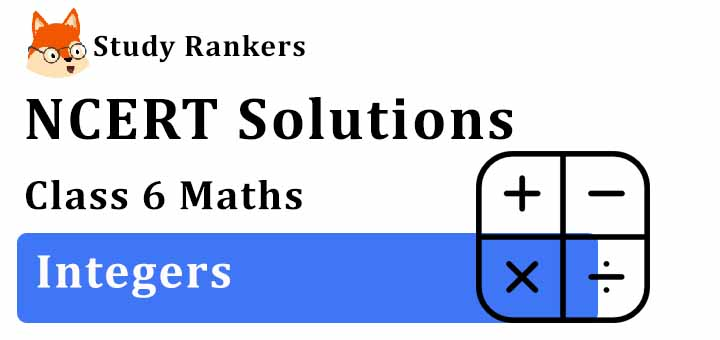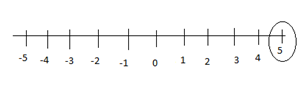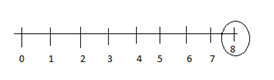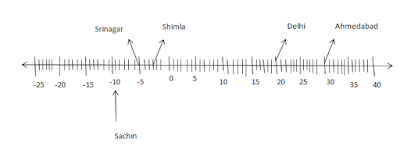# NCERT Solutions for Class 6 Maths Chapter 6 Integers

In this page, you will get Chapter 6 Integers NCERT Solutions for Class 6 Maths which will make learning exciting and cover the syllabus in less time. Class 6 Maths NCERT Solutions will helpful in understanding the key concepts of the chapter properly. These NCERT Solutions are helpful resources that can help you not only cover the entire syllabus but also help in solving the difficulties that lie ahead with ease.

Chapter 6 NCERT Solutions will help you in improving the marks in the examinations and have edge over your classmates. It is very challenging to score good marks in tests that is why we have prepared NCERT Solutions.Exercise 6.1

1. Write opposites of the following :
(a) Increase in weight
(b) 30 km north
(c) 326 BC
(d) Loss of Rs 700
(e) 100 m above sea level

(a) Decrease in weight
(b) 30 km south
(c) 326 A.D.
(d) Gain of Rs 700

(e) 100 m below sea level

2. Represent the following numbers as integers with appropriate signs.
(a) An aeroplane is flying at a height two thousand metre above the ground.
(b) A submarine is moving at a depth, eight hundred metre below the sea level.
(c) A deposit of rupees two hundred.
(d) Withdrawal of rupees seven hundred.

(a) + 2000
(b) – 800
(c) + 200
(d) – 700

3. Represent the following numbers on a number line :
(a) + 5
(b) – 10
(c) + 8
(d) – 1
(e) – 6

(a)(b)(c)(d)(e)4. Adjacent figure is a vertical number line, representing integers. Observe it and locate the following points :
(a) If point D is + 8, then which point is – 8?
(b) Is point G a negative integer or a positive integer?
(c) Write integers for points B and E.
(d) Which point marked on this number line has the least value?
(e) Arrange all the points in decreasing order of value(a) F
(b) Negative integer -6
(c) Point B represents 4 and point E represents – 10
(d) E has the least value as it represent – 10
(e) D > C > B > A > O > H > G > F > E

5. Following is the list of temperatures of five places in India on a particular day of the year.
Place Temperature
Sachin 10°C below 0°C .................
Shimla 2°C below 0°C .................
Delhi 20°C above 0°C .................
Srinagar 5°C below 0°C .................(a) Write the temperatures of these places in the form of integers in the blank column.
(b) Following is the number line representing the temperature in degree CelsiusPlot the name of the city against its temperature.
(c) Which is the coolest place?
(d) Write the names of the places where temperatures are above 10°C

a) Sachin → – 10°C
Shimla → -2°C
Delhi → +20°C
Srinagar → -5°C
b)c) Sachin

6. In each of the following pairs, which number is to the right of the other on the number line?
(a) 2, 9
(b) – 3, – 8
(c) 0, – 1
(d) – 11, 10
(e) – 6, 6
(f) 1, – 100

a) 9(9 >2)
b) -3 (-3 > -8 )
c) 0 ( 0 > -1)
d) 10(10 > -11)
e) 6 (6 > -6 )
f) 1(1 > -100)

7. Write all the integers between the given pairs (write them in the increasing order.)
(a) 0 and – 7
(b) – 4 and 4
(c) – 8 and – 15
(d) – 30 and – 23

a) -6 , -5 , -4 , -3 , -2 ,- 1
b) -3, -2 ,-1 , 0 , 1 , 2 , 3
c) -14 , -13 , -12 , -11 ,-10 ,-9
d) -29, -28 , -27 , -26 , -25 ,-24

8. (a) Write four negative integers greater than – 20.
(b) Write four negative integers less than – 10

a) -19 ,- 18 , -17 , -16
b) -11 , -12, -13 , 14

9. For the following statements, write True (T) or False (F).
If the statement is false, correct the statement.
(a) – 8 is to the right of – 10 on a number line.
(b) – 100 is to the right of – 50 on a number line.
(c) Smallest negative integer is – 1.
(d) – 26 is greater than – 25.

a) True
b) False (-50 > -100) -100 is to the left of -50 on a number line
c) False , -1 is greatest integer
d) False , -26 is smaller than -25

10. Draw a number line and answer the following :
(a) Which number will we reach if we move 4 numbers to the right of – 2.
(b) Which number will we reach if we move 5 numbers to the left of 1.
(c) If we are at – 8 on the number line, in which direction should we move to reach – 13?
(d) If we are at – 6 on the number line, in which direction should we move to reach – 1?

a)We move 4 numbers to the right of -2.
b) We move 5 numbers to the left of 1 .c)13 is to the left of -8
d)-1 is to the right of -6

Exercise 6.2

1. Using the number line write the integer which is :
(a) 3 more than 5
(b) 5 more than –5
(c) 6 less than 2

(d) 3 less than –2Exercise 6.3

1. Find
(a) 35 – (20)
(b) 72 – (90)
(c) (– 15) – (– 18)
(d) (–20) – (13)
(e) 23 – (– 12)
(f) (–32) – (– 40)

(a) 35 – (20) = 15
(b) 72 – (90) = -18
(c) (– 15) – (– 18) = -15 +18 = 3
(d) (–20) – (13) = -33
(e) 23 – (– 12) = 23 + 12 = 35
(f) (–32) – (– 40) = -32 + 40 = 8

2. Fill in the blanks with >, < or = sign.
(a) (– 3) + (– 6) ______ (– 3) – (– 6)
(b) (– 21) – (– 10) _____ (– 31) + (– 11)
(c) 45 – (– 11) ______ 57 + (– 4)
(d) (– 25) – (– 42) _____ (– 42) – (– 25)

(a) (– 3) + (– 6) = -9
(– 3) – (-6) = -3 + 6 = 3
-9 < 3

(b) (– 21) – (– 10) = -21 + 10 = -11
-31 + (-11) = -42
-11 > -42

(c) 45 – (– 11) = 45 + 11 = 56
57 + (-4) = 57 – 4 = 53
56 > 53

(d) (– 25) – (– 42) = -25 + 42 = 17
- 42 – (-25) = -42 + 25 = -17
17 > -17

3. Fill in the blanks.
(a) (– 8) + _____ = 0
(b) 13 + _____ = 0
(c) 12 + (– 12) = ____
(d) (– 4) + ____ = – 12
(e) ____ – 15 = – 10

(a) -8 + (-8) = 0
(b) 13 + (-13) = 0
(c) 12 + (– 12) = 0
(d) (– 4) + (-8) = -12
(e) -5 – 15 = -10

4. Find
(a) (– 7) – 8 – (– 25)
(b) (– 13) + 32 – 8 – 1
(c) (– 7) + (– 8) + (– 90)
(d) 50 – (– 40) – (– 2)

(a) (– 7) – 8 – (–25)
= -7 – 8 + 25 = - 15 + 25 = 10

(b) (– 13) + 32 – 8 – 1
= - 13 + 32 – 8 – 1 = 32 – 22 = 10

(c) (-7) +(-8) +(-90)
= -7 -8 -90 = -105

(d) 50 – (– 40) – (–2)
= 50 + 40 + 2 = 92

## NCERT Solutions for Class 6 Maths Chapter 6 Integers

NCERT Solutions of Chapter 6 Integers will help in developing basic problem solving skills The collection of the numbers, that is, … -4, -3, -2, -1, 0, 1, 2, 3, 4,….is called integers.

• The numbers -1, -2, -3, -4… which are called negative numbers, are also called negative integers.

• The number 1, 2, 3, 4 …s, which are called positive numbers, are also called positive integers.

• When two positive integers are added, then we get an integer with a positive sign. When two negative integers are added, then we get an integer with a negative sign.

• When we subtract a larger positive integer from a smaller positive integer, the difference is a negative integer.

You will get exercisewise NCERT Solutions for Class 6 which will make you well versed in variety of topics and you will be able to instantly recollect your ideas and shape your answers. It will make much easier to memorize topics faster and frame better answers.

• Exercise 6.1 Chapter 6 Class 6 Maths NCERT Solutions
• Exercise 6.2 Chapter 6 Class 6 Maths NCERT Solutions
• Exercise 6.3 Chapter 6 Class 6 Maths NCERT Solutions

Through the help of the concepts, you can easily tackle the questions given the supplementary books. NCERT Solutions will help in building a great foundation of concepts and make easy for the students to understand basics.

### NCERT Solutions for Class 6 Maths Chapters:

 Chapter 1 Knowing Our Numbers Chapter 2 Whole Numbers Chapter 3 Playing with Numbers Chapter 4 Basic Geometrical Ideas Chapter 5 Understanding Elementary Shapes Chapter 7 Fractions Chapter 8 Decimals Chapter 9 Data Handling Chapter 10 Mensuration Chapter 11 Algebra Chapter 12 Ratio and Proportion Chapter 13 Symmetry Chapter 14 Practical Geometry

FAQ on Chapter 6 Integers

#### How many exercises in Chapter 6 Integers?

There are only three exercises in the Chapter 6 Integers which will help the students in learning complex topics and problems in an easy way. These NCERT Solutions are prepared as per the accordance of latest CBSE guidelines so you can score maximum marks.

#### Find the value of :(–7) + (–9) + 4 + 16.

(–7) + (–9) + 4 + 16
= (–16) + 20
= (–16) + 16 + 4
= 0 + 4 = 4.

#### Find the solution of the following :(–9) + (+13)

(–9) + (+13)
= (–9) + (+9) + (+4)
= 0 + (+4) = +4

#### Write all the integers between -8 and -15 in the increasing order.

The integers between –8 and –15 in increasing order are –14, –13, –12, –11, –10 and –9.